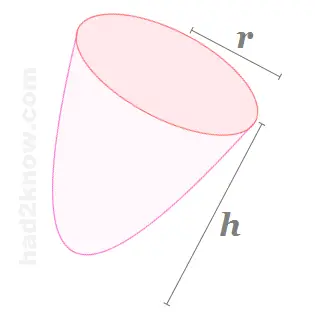# Paraboloid Surface Area and Volume Calculator

Paraboloid Calculator
Height =

A paraboloid is a solid of revolution that results from rotating a parabola around its axis of symmetry. If you know the height and radius of a paraboloid, you can compute its volume and surface area with simple geometry formulas. You can also use the calculator on the left.### Volume Formula

If the height of a paraboloid is denoted by h and the radius by r, then the volume is given by the equation

V = (π/2)hr²

Notice that this is less than the volume of a cylinder but more than the volume of a cone with the same dimensions.

### Surface Area Formula

The surface area of a paraboloid, not including its base, is given by the formula

SA = (π/6)(r/h²)[(r² + 4h²)3/2 - r³]

Since the base of the figure has an area of πr², the formula for surface area including the base is

SAb = (π/6)(r/h²)[(r² + 4h²)3/2 - r³] + π